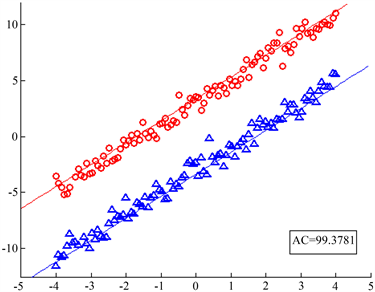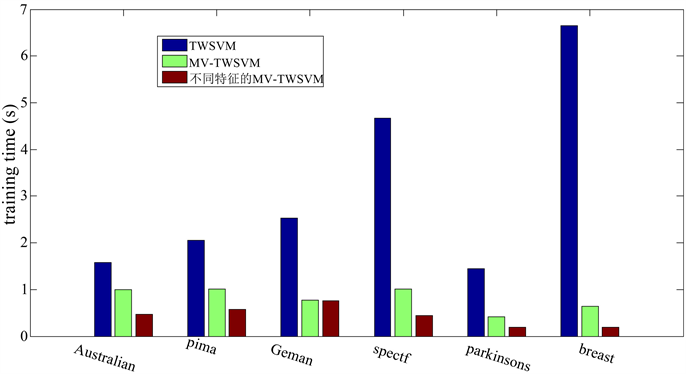﻿ 基于多视图双支持向量机半监督学习机

基于多视图双支持向量机半监督学习机Semi-Supervised Learning Machine Based on Multi-View Twin Support Vector Machine

Abstract: In many practical problems of machine learning, the data has multi-views; multi-views complement each other; and the classification effect is better. This paper mainly studies the semi-supervised method of multi-view dual support vector machine, and divides the data according to different characteristics. Multi-views are used to find two non-parallel hyperplanes for each view, and the model is constructed to solve the dual problem. Experimental results show that the proposed algorithm can reduce the dimension of the data and has good classification accuracy. The support vector machine algorithm shortens the running time, reduces the computational complexity, and predicts better performance.

1. 引言

2. 相关工作

2.1. 支持向量机

$f\left(x\right)={w}^{T}x+b=0,$ (1)

$\begin{array}{ll}\underset{w,b,\xi }{\mathrm{min}}\text{ }\hfill & \frac{1}{2}{\left(‖Aw+{e}_{1}b‖}^{2}+c{e}_{2}^{T}\xi ,\hfill \\ \text{s}\text{.t}\text{.}\text{ }\hfill & -\left(Bw+{e}_{2}b\right)\ge {e}_{2}-\xi ,\hfill \\ \hfill & \xi \ge 0,\hfill \end{array}$ (2)

2.2. 线性可分的双支持向量机

${f}_{1}\left(x\right)={w}_{1}^{T}x+{b}_{1}=0,{f}_{2}\left(x\right)={w}_{2}^{T}x+{b}_{2}=0,$ (3)

$\begin{array}{ll}\text{TWSVMM}1:\underset{{w}_{1},{b}_{1},\xi }{\mathrm{min}}\hfill & \frac{1}{2}{\left(‖A{w}_{1}+{e}_{1}{b}_{1}‖}^{2}+{c}_{1}{e}_{2}^{T}\xi ,\hfill \\ \text{s}\text{.t}\text{.}\text{ }\hfill & -\left(B{w}_{1}+{e}_{2}{b}_{1}\right)\ge {e}_{2}-\xi \hfill \\ \hfill & \xi \ge 0,\hfill \end{array}$ (4)

$\begin{array}{ll}\text{TWSVMM}2:\underset{{w}_{2},{b}_{2},\xi }{\mathrm{min}}\hfill & \frac{1}{2}{\left(‖B{w}_{2}+{e}_{2}{b}_{2}‖}^{2}+{c}_{2}{e}_{1}^{T}\xi ,\hfill \\ \text{s}\text{.t}\text{.}\text{ }\hfill & A{w}_{2}+{e}_{1}{b}_{2}\ge {e}_{1}-\xi ,\hfill \\ \hfill & \xi \ge 0,\hfill \end{array}$ (5)

$f\left(x\right)=\mathrm{arg}\underset{k=1,2}{\mathrm{min}}\frac{|{w}_{k}^{T}x+{b}_{k}|}{‖{w}_{k}‖},$ (6)

2.3. 线性不可分的双支持向量机

${g}_{1}\left(x\right)=K\left({x}^{T},{C}^{T}\right){u}_{1}+{b}_{1}=0,{g}_{2}\left(x\right)=K\left({x}^{T},{C}^{T}\right){u}_{2}+{b}_{2}=0,$ (7)

$\begin{array}{ll}\text{KTWSVM}1:\underset{{u}_{1},{b}_{1},\xi }{\mathrm{min}}\hfill & \frac{1}{2}{\left(‖K\left(A,{C}^{T}\right){u}_{1}+{e}_{1}{b}_{1}‖\right)}^{2}+{c}_{1}{e}_{2}^{T}\xi ,\hfill \\ \text{s}\text{.t}\text{.}\text{ }\hfill & -\left[K\left(B,{C}^{T}\right){u}_{1}+{e}_{2}{b}_{1}\right]\ge {e}_{2}-\xi ,\hfill \\ \hfill & \xi \ge 0,\hfill \end{array}$ (8)

$\begin{array}{ll}\text{TWSVM}2:\underset{{u}_{2},{b}_{2},\xi }{\mathrm{min}}\hfill & \frac{1}{2}{\left(‖K\left(B,{C}^{T}\right){u}_{2}+{e}_{2}{b}_{2}‖}^{2}+{c}_{2}{e}_{1}^{T}\xi ,\hfill \\ \text{s}\text{.t}\text{.}\text{ }\hfill & K\left(A,{C}^{T}\right){u}_{2}+{e}_{1}{b}_{2}\ge {e}_{1}-\xi ,\hfill \\ \hfill & \xi \ge 0,\hfill \end{array}$ (9)

2.4. 半监督多视图学习

$\begin{array}{cc}f=\mathrm{arg}\mathrm{min}& \underset{i=1}{\overset{l}{\sum }}{\left[{y}_{i}-{f}^{\left(1\right)}\left({x}_{i}^{\left(1\right)}\right)\right]}^{2}+\mu \underset{i=1}{\overset{l}{\sum }}{\left[{y}_{i}-{f}^{\left(2\right)}\left({x}_{i}^{\left(2\right)}\right)\right]}^{2}+{\gamma }_{1}{‖{f}^{\left(1\right)}‖}^{2}\\ & +{\gamma }_{2}{‖{f}^{\left(2\right)}‖}^{2}+\frac{\gamma C}{l+u}\underset{i=1}{\overset{l+u}{\sum }}{\left[{f}^{\left(1\right)}\left({x}_{i}^{\left(1\right)}\right)-{f}^{\left(2\right)}\left({x}_{i}^{\left(2\right)}\right)\right]}^{2},\end{array}$ (10)

$f=\mathrm{min}\left[\underset{i=1}{\overset{l+u}{\sum }}{\alpha }_{i}{K}^{\left(1\right)}\left({x}^{\left(1\right)},{x}_{i}^{\left(1\right)}\right),\underset{i=1}{\overset{l+u}{\sum }}{\beta }_{i}{K}^{\left(2\right)}\left({x}^{\left(2\right)},{x}_{i}^{\left(2\right)}\right)\right],i=1,2,\cdots ,l+u$ (11)

$\begin{array}{l}\left[\frac{1}{l}J{K}_{1}+{\gamma }_{1}I+\frac{\gamma C}{l+u}{K}_{1}\right]\alpha -\frac{\gamma C}{l+u}{K}_{2}\beta =\frac{1}{l}Y\right],\\ \left[\frac{u}{l}J{K}_{2}+{\gamma }_{2}I+\frac{\gamma C}{l+u}{K}_{2}\right]\left[\beta -\frac{\gamma C}{l+u}{K}_{1}\alpha =\frac{u}{l}Y\right],\end{array}$ (12)

3. 基于多视图双支持向量机的半监督学习方法

$\begin{array}{l}f\left(x\right)=\mathrm{min}\left\{{f}_{1}\left(x\right),{f}_{2}\left(x\right)\right\}\\ \text{ }\text{ }\text{\hspace{0.17em}}=\mathrm{min}\left\{\mathrm{min}|{w}^{\left(1i\right)T}x+{b}^{\left(1i\right)}|,\mathrm{min}|{w}^{\left(2i\right)T}x+{b}^{\left(2i\right)}|\right\},\\ i=1,2,\end{array}$

$\begin{array}{l}\begin{array}{cc}\text{MV-TWSVM}1:& \mathrm{min}\end{array}\text{ }\frac{1}{2}\left({‖{A}_{1}{w}^{\left(11\right)}+{e}_{1}{b}^{\left(11\right)}‖}^{2}+{‖{A}_{2}{w}^{\left(12\right)}+{e}_{1}{b}^{\left(12\right)}‖}^{2}\right)+{c}_{11}{e}_{2}^{T}{\xi }^{\left(11\right)}+{c}_{12}{e}_{2}^{T}{\xi }^{\left(12\right)}\\ \text{ }\text{ }\text{ }\text{ }\text{ }\text{ }\text{ }\text{ }\text{ }\text{\hspace{0.17em}}+\frac{1}{2}{\gamma }_{1}{c}_{1}{‖\left({U}_{1}{w}^{\left(11\right)}+{e}_{3}{b}^{\left(11\right)}\right)-\left({U}_{2}{w}^{\left(12\right)}+{e}_{3}{b}^{\left(12\right)}\right)‖}^{2},\\ \text{ }\text{ }\text{ }\text{ }\text{ }\text{ }\text{ }\text{\hspace{0.17em}}\text{s}\text{.t}\text{.}\text{ }-\left({B}_{1}{w}^{\left(11\right)}+{e}_{2}{b}^{\left(11\right)}\right)\ge {e}_{2}-{\xi }^{\left(11\right)},\\ \text{ }\text{ }\text{ }\text{ }\text{ }\text{ }\text{ }\text{ }\text{ }\text{\hspace{0.17em}}-\left({B}_{2}{w}^{\left(12\right)}+{e}_{2}{b}^{\left(12\right)}\right)\ge {e}_{2}-{\xi }^{\left(12\right)},\\ \text{ }\text{ }\text{ }\text{ }\text{ }\text{ }\text{ }\text{ }\text{ }\text{\hspace{0.17em}}{\xi }^{\left(11\right)}\ge 0,{\xi }^{\left(12\right)}\ge 0,\end{array}$ (13)

$\begin{array}{l}\begin{array}{cc}\text{MV-TWSVM2}:& \mathrm{min}\end{array}\text{ }\frac{1}{2}\left({‖{B}_{1}{w}^{\left(21\right)}+{e}_{2}{b}^{\left(21\right)}‖}^{2}+{‖{B}_{2}{w}^{\left(22\right)}+{e}_{2}{b}^{\left(22\right)}‖}^{2}\right)+{c}_{21}{e}_{1}^{T}{\xi }^{\left(21\right)}+{c}_{22}{e}_{2}^{T}{\xi }^{\left(22\right)}\\ \text{ }\text{ }\text{ }\text{ }\text{ }\text{ }\text{ }\text{ }\text{ }\text{\hspace{0.17em}}+\frac{1}{2}{\gamma }_{2}{c}_{2}{‖\left({U}_{1}{w}^{\left(21\right)}+{e}_{3}{b}^{\left(21\right)}\right)-\left({U}_{2}{w}^{\left(22\right)}+{e}_{3}{b}^{\left(22\right)}\right)‖}^{2},\\ \text{ }\text{ }\text{ }\text{ }\text{ }\text{ }\text{ }\text{\hspace{0.17em}}\text{s}\text{.t}\text{.}\text{ }\left({A}_{1}{w}^{\left(21\right)}+{e}_{1}{b}^{\left(21\right)}\right)\ge {e}_{1}-{\xi }^{\left(21\right)},\\ \text{ }\text{ }\text{ }\text{ }\text{ }\text{ }\text{ }\text{ }\text{ }\text{\hspace{0.17em}}\left({A}_{2}{w}^{\left(22\right)}+{e}_{1}{b}^{\left(22\right)}\right)\ge {e}_{1}-{\xi }^{\left(22\right)},\\ \text{ }\text{ }\text{ }\text{ }\text{ }\text{ }\text{ }\text{ }\text{ }\text{\hspace{0.17em}}{\xi }^{\left(21\right)}\ge 0,{\xi }^{\left(22\right)}\ge 0,\end{array}$ (14)

$\begin{array}{l}L\left({w}^{\left(11\right)},{w}^{\left(12\right)},{b}^{\left(11\right)},{b}^{\left(12\right)},{\alpha }_{11},{\alpha }_{12},{\beta }_{11},{\beta }_{12}\right)\\ =\frac{1}{2}{\left({A}_{1}{w}^{\left(11\right)}+{e}_{1}{b}^{\left(11\right)}\right)}^{T}\left({A}_{1}{w}^{\left(11\right)}+{e}_{1}{b}^{\left(11\right)}\right)+\frac{1}{2}{\left({A}_{2}{w}^{\left(12\right)}+{e}_{1}{b}^{\left(12\right)}\right)}^{T}\left({A}_{2}{w}^{\left(12\right)}+{e}_{1}{b}^{\left(12\right)}\right)\\ +\frac{1}{2}{\left[\left({U}_{1}{w}^{\left(11\right)}+{e}_{3}{b}^{\left(11\right)}\right)-\left({U}_{2}{w}^{\left(12\right)}+{e}_{3}{b}^{\left(12\right)}\right)\right]}^{T}\left[\left({U}_{1}{w}^{\left(11\right)}+{e}_{3}{b}^{\left(11\right)}\right)-\left({U}_{2}{w}^{\left(12\right)}+{e}_{3}{b}^{\left(12\right)}\right)\right]\\ +{\alpha }_{11}^{T}\left[{B}_{1}{w}^{\left(11\right)}+{e}_{2}{b}^{\left(11\right)}+{e}_{2}-{\xi }^{\left(11\right)}\right]+{\alpha }_{12}^{T}\left[{B}_{2}{w}^{\left(12\right)}+{e}_{2}{b}^{\left(12\right)}+{e}_{2}-{\xi }^{\left(12\right)}\right]\\ +{c}_{11}{e}_{2}^{T}{\xi }^{\left(11\right)}+{c}_{12}{e}_{2}^{T}{\xi }^{\left(12\right)}-{\beta }_{11}^{T}{\xi }^{\left(11\right)}-{\beta }_{12}^{T}{\xi }^{\left(12\right)},\end{array}$ (15)

$L$ 的分量求导并令其为0得：

${\nabla }_{{w}^{\left(11\right)}}L:\text{ }{A}_{1}^{T}\left({A}_{1}{w}^{\left(11\right)}+{e}_{1}{b}^{\left(11\right)}\right)+{\gamma }_{1}{c}_{1}{U}_{1}^{T}\left[\left({U}_{1}{w}^{\left(11\right)}+{e}_{3}{b}^{\left(11\right)}\right)-\left({U}_{2}{w}^{\left(12\right)}+{e}_{3}{b}^{\left(12\right)}\right)\right]+{B}_{1}^{T}{\alpha }_{11}=0,$ (16)

${\nabla }_{{w}^{\left(12\right)}}L:\text{ }{A}_{2}^{T}\left({A}_{2}{w}^{\left(12\right)}+{e}_{1}{b}^{\left(11\right)}\right)+{\gamma }_{1}{c}_{1}{U}_{2}^{T}\left[\left({U}_{1}{w}^{\left(11\right)}+{e}_{3}{b}^{\left(11\right)}\right)-\left({U}_{2}{w}^{\left(12\right)}+{e}_{3}{b}^{\left(12\right)}\right)\right]+{B}_{2}^{T}{\alpha }_{12}=0,$ (17)

${\nabla }_{{b}^{\left(11\right)}}L\text{\hspace{0.17em}}:\text{ }{e}_{1}^{T}\left({A}_{1}{w}^{\left(11\right)}+{e}_{1}{b}^{\left(11\right)}\right)+{\gamma }_{1}{c}_{1}{e}_{3}^{T}\left[\left({U}_{1}{w}^{\left(11\right)}+{e}_{3}{b}^{\left(11\right)}\right)-\left({U}_{2}{w}^{\left(12\right)}+{e}_{3}{b}^{\left(12\right)}\right)\right]+{e}_{2}^{T}{\alpha }_{11}=0,$ (18)

${\nabla }_{{b}^{\left(12\right)}}L:\text{ }{e}_{1}^{T}\left({A}_{2}{w}^{\left(12\right)}+{e}_{1}{b}^{\left(12\right)}\right)-{\gamma }_{1}{c}_{1}{e}_{3}^{T}\left[\left({U}_{1}{w}^{\left(11\right)}+{e}_{3}{b}^{\left(11\right)}\right)-\left({U}_{2}{w}^{\left(12\right)}+{e}_{3}{b}^{\left(12\right)}\right)\right]+{e}_{2}^{T}{\alpha }_{12}=0,$ (19)

${\nabla }_{{\xi }^{\left(11\right)}}L:{c}_{11}{e}_{2}^{T}-{\alpha }_{11}^{T}-{\beta }_{11}=0,$ (20)

${\nabla }_{{\xi }^{\left(12\right)}}L:{c}_{12}{e}_{2}^{T}-{\alpha }_{12}^{T}-{\beta }_{12}=0,$ (21)

${\nabla }_{{\alpha }_{11}}L:\text{\hspace{0.17em}}{\alpha }_{11}^{T}\left({B}_{1}{w}^{\left(11\right)}+{e}_{2}{b}^{\left(11\right)}-{\xi }^{\left(11\right)}\right)=0,$ (22)

${\nabla }_{{\alpha }_{12}}L:\text{\hspace{0.17em}}{\alpha }_{12}^{T}\left({B}_{2}{w}^{\left(12\right)}+{e}_{2}{b}^{\left(12\right)}-{\xi }^{\left(12\right)}\right)=0,$ (23)

${\nabla }_{{\beta }_{11}}L:{\beta }_{11}^{T}{\xi }^{\left(11\right)}=0,$ (24)

${\nabla }_{{\beta }_{12}}L:{\beta }_{12}^{T}{\xi }^{\left(12\right)}=0,$ (25)

$-\left({B}_{1}{w}^{\left(11\right)}+{e}_{2}{b}^{\left(11\right)}\right)+{\xi }^{\left(11\right)}\ge {e}_{2},$ (26)

$-\left({B}_{2}{w}^{\left(11\right)}+{e}_{2}{b}^{\left(12\right)}\right)+{\xi }^{\left(12\right)}\ge {e}_{2},$ (27)

$\left(\left[\begin{array}{c}{A}_{1}^{T}\\ {e}_{1}^{T}\end{array}\right]\left[\begin{array}{cc}{A}_{1}& {e}_{1}\end{array}\right]+{c}_{1}{\gamma }_{1}\left[\begin{array}{c}{u}_{1}^{T}\\ {e}_{3}^{T}\end{array}\right]\left[\begin{array}{cc}{u}_{1}& {e}_{3}\end{array}\right]\right)\left[\begin{array}{c}{w}^{\left(11\right)}\\ {b}^{\left(11\right)}\end{array}\right]-{c}_{1}{\gamma }_{1}\left[\begin{array}{c}{u}_{1}^{T}\\ {e}_{3}^{T}\end{array}\right]\left[\begin{array}{cc}{u}_{2}& {e}_{3}\end{array}\right]\left[\begin{array}{c}{w}^{\left(12\right)}\\ {b}^{\left(12\right)}\end{array}\right]+{\alpha }_{11}\left[\begin{array}{c}{B}_{1}^{T}\\ {e}_{2}^{T}\end{array}\right]{\alpha }_{12}=0,$ (28)

$-{c}_{1}{\gamma }_{1}\left[\begin{array}{c}{U}_{2}^{T}\\ {e}_{3}^{T}\end{array}\right]\left[\begin{array}{cc}{U}_{1}& {e}_{3}\end{array}\right]\left[\begin{array}{c}{w}^{\left(11\right)}\\ {b}^{\left(11\right)}\end{array}\right]+\left(\left[\begin{array}{c}{A}_{2}^{T}\\ {e}_{1}^{T}\end{array}\right]\left[\begin{array}{cc}{A}_{2}& {e}_{1}\end{array}\right]+{c}_{1}{\gamma }_{1}\left[\begin{array}{c}{U}_{2}^{T}\\ {e}_{3}^{T}\end{array}\right]\left[\begin{array}{cc}{U}_{2}& {e}_{3}\end{array}\right]\right)\left[\begin{array}{c}{w}^{\left(12\right)}\\ {b}^{\left(12\right)}\end{array}\right]+\left[\begin{array}{c}{B}_{2}^{T}\\ {e}_{2}^{T}\end{array}\right]{\alpha }_{12}=0,$ (29)

${H}_{1}=\left[{A}_{1}\text{ }{e}_{1}\right],{H}_{2}=\left[{A}_{2}\text{ }{e}_{1}\right],$

${P}_{1}=\left[{U}_{1}\text{ }{e}_{3}\right],{P}_{2}=\left[{U}_{2}\text{ }{e}_{3}\right],$

${G}_{1}={\left[{B}_{1}\text{ }{e}_{2}\right]}^{T},{G}_{2}={\left[{B}_{2}\text{ }{e}_{2}\right]}^{T},$

$\begin{array}{l}H={H}_{1}^{T}{H}_{1}+{c}_{1}{\gamma }_{1}{P}_{1}^{T}{P}_{1},F=-{c}_{1}{\gamma }_{1}{P}_{1}^{T}{P}_{2},\\ P={H}_{2}^{T}{H}_{2}+{c}_{1}{\gamma }_{1}{P}_{2}^{T}{P}_{2},Q={F}^{-1}H-{P}^{-1}{F}^{T},\\ {u}_{1}={\left[{w}^{\left(11\right)}\text{ }{b}^{\left(11\right)}\right]}^{T},{u}_{2}={\left[{w}^{\left(12\right)}\text{ }{b}^{\left(12\right)}\right]}^{T},\end{array}$ (30)

$H{u}_{1}+F{u}_{2}=-{G}_{1}{\alpha }_{11},$ (31)

${F}^{T}{u}_{1}+P{u}_{2}=-{G}_{2}{\alpha }_{12},$ (32)

${u}_{1}=-{Q}^{-1}{F}^{-1}{G}_{1}{\alpha }_{11}+{Q}^{-1}{P}^{-1}{G}_{2}{\alpha }_{12},$ (33)

${u}_{2}=-Q{H}^{-1}{G}_{1}{\alpha }_{11}+Q{\left({F}^{T}\right)}^{-1}{G}_{2}{\alpha }_{12},$ (34)

$\begin{array}{cc}\mathrm{max}& \frac{1}{2}{\alpha }_{1}^{T}M{\alpha }_{1}+{e}_{2}^{T}{\alpha }_{1},\\ \text{s}\text{.t}.& 0\le {\alpha }_{1}\le {c}_{1},\end{array}$ (35)

$M=\left(\begin{array}{cc}{M}_{1}& {M}_{2}\\ {M}_{3}& {M}_{4}\end{array}\right),{\alpha }_{1}=\left(\begin{array}{c}{\alpha }_{11}\\ {\alpha }_{12}\end{array}\right),{c}_{1}=\left(\begin{array}{c}{c}_{11}\\ {c}_{12}\end{array}\right),$

${M}_{1}={M}_{11}^{T}H{M}_{11}+{M}_{13}^{T}P{M}_{13}-{M}_{11}^{T}F{M}_{13}-{M}_{13}^{T}{F}^{T}{M}_{11}-2{G}_{1}^{T}{M}_{11},$

${M}_{2}={M}_{12}^{T}H{M}_{12}+{M}_{14}^{T}P{M}_{14}-{M}_{12}^{T}F{M}_{14}-{M}_{14}^{T}{F}^{T}{M}_{12}-2{G}_{2}^{T}{M}_{12},$

${M}_{3}=-{M}_{11}^{T}H{M}_{12}-{M}_{13}^{T}P{M}_{14}+{M}_{11}^{T}F{M}_{14}+{M}_{13}^{T}{F}^{T}{M}_{12}-2{G}_{1}^{T}{M}_{12},$

${M}_{4}=-{M}_{12}^{T}H{M}_{11}-{M}_{14}^{T}P{M}_{13}+{M}_{12}^{T}F{M}_{13}+{M}_{14}^{T}{F}^{T}{M}_{11}-2{G}_{2}^{T}{M}_{13},$

${M}_{11}={Q}^{-1}{F}^{-1}{G}_{1},{M}_{12}={Q}^{-1}{F}^{-1}{G}_{2},$

${M}_{13}=Q{H}^{-1}{G}_{1},{M}_{14}=Q{\left({F}^{T}\right)}^{-1}{G}_{2},$

$\left(\left[\begin{array}{c}{B}_{1}^{T}\\ {e}_{2}^{T}\end{array}\right]\left[\begin{array}{cc}{B}_{1}& {e}_{2}\end{array}\right]+{c}_{2}{\gamma }_{2}\left[\begin{array}{c}{U}_{1}^{T}\\ {e}_{3}^{T}\end{array}\right]\left[\begin{array}{cc}{U}_{1}& {e}_{3}\end{array}\right]\right)\left[\begin{array}{c}{w}^{\left(21\right)}\\ {b}^{\left(21\right)}\end{array}\right]-{c}_{2}{\gamma }_{2}\left[\begin{array}{c}{U}_{1}^{T}\\ {e}_{3}^{T}\end{array}\right]\left[\begin{array}{cc}{U}_{2}& {e}_{3}\end{array}\right]\left[\begin{array}{c}{w}^{\left(22\right)}\\ {b}^{\left(22\right)}\end{array}\right]+{\alpha }_{21}\left[\begin{array}{c}{A}_{1}^{T}\\ {e}_{1}^{T}\end{array}\right]=0,$ (36)

$-{c}_{2}{\gamma }_{2}\left[\begin{array}{c}{U}_{2}^{T}\\ {e}_{3}^{T}\end{array}\right]\left[\begin{array}{cc}{U}_{1}& {e}_{3}\end{array}\right]\left[\begin{array}{c}{w}^{\left(21\right)}\\ {b}^{\left(21\right)}\end{array}\right]+\left(\left[\begin{array}{c}{B}_{2}^{T}\\ {e}_{2}^{T}\end{array}\right]\left[\begin{array}{cc}{B}_{2}& {e}_{2}\end{array}\right]+{c}_{2}{\gamma }_{2}\left[\begin{array}{c}{U}_{2}^{T}\\ {e}_{3}^{T}\end{array}\right]\left[\begin{array}{cc}{U}_{2}& {e}_{3}\end{array}\right]\right)\left[\begin{array}{c}{w}^{\left(22\right)}\\ {b}^{\left(22\right)}\end{array}\right]+\left[\begin{array}{c}{A}_{2}^{T}\\ {e}_{1}^{T}\end{array}\right]{\alpha }_{22}=0$ (37)

$\begin{array}{l}X={G}_{1}{}^{T}{G}_{1}+{c}_{2}{\gamma }_{2}{P}_{1}^{T}{P}_{1},Y=-{c}_{2}{\gamma }_{2}{P}_{1}^{T}{P}_{2},\\ R={G}_{2}{}^{T}{G}_{2}+{c}_{2}{\gamma }_{2}{P}_{2}^{T}{P}_{2},S={Y}^{-1}X-{R}^{-1}{Y}^{T},\\ {v}_{1}={\left[}^{{w}^{\left(21\right)}},{v}_{2}={\left[}^{{w}^{\left(22\right)}},\end{array}$ (38)

${v}_{1}={S}^{-1}{Y}^{-1}{H}_{1}{\alpha }_{21}+{S}^{-1}{R}^{-1}{H}_{2}{\alpha }_{22},$ (39)

${v}_{2}=S{X}^{-1}{H}_{1}{\alpha }_{21}+S{\left({Y}^{T}\right)}^{-1}{H}_{2}{\alpha }_{22},\text{\hspace{0.17em}}$ (40)

$\begin{array}{cc}\mathrm{max}& \frac{1}{2}{\alpha }_{2}^{T}N{\alpha }_{2}+{e}_{1}^{T}{\alpha }_{2},\\ \text{s}\text{.t}.& 0\le {\alpha }_{2}\le {c}_{2},\end{array}$ (41)

$N=\left(\begin{array}{cc}{N}_{1}& {N}_{2}\\ {N}_{3}& {N}_{4}\end{array}\right),{\alpha }_{2}=\left(\begin{array}{c}{\alpha }_{21}\\ {\alpha }_{22}\end{array}\right),{c}_{2}=\left(\begin{array}{c}{c}_{21}\\ {c}_{22}\end{array}\right),$

${N}_{1}={M}_{21}^{T}X{M}_{21}+{M}_{23}^{T}R{M}_{23}-{M}_{21}^{T}Y{M}_{23}-{M}_{23}^{T}{Y}^{T}{M}_{21}-2{G}_{1}^{T}{M}_{21},$

${N}_{2}={M}_{22}^{T}X{M}_{22}+{M}_{24}^{T}R{M}_{24}-{M}_{22}^{T}Y{M}_{24}-{M}_{24}^{T}{Y}^{T}{M}_{22}-2{G}_{2}^{T}{M}_{22},$

${N}_{3}=-{M}_{21}^{T}X{M}_{22}-{M}_{23}^{T}R{M}_{24}+{M}_{21}^{T}Y{M}_{24}+{M}_{23}^{T}{Y}^{T}{M}_{22}-2{G}_{1}^{T}{M}_{22},$

${N}_{4}=-{M}_{22}^{T}X{M}_{21}-{M}_{24}^{T}R{M}_{23}+{M}_{22}^{T}Y{M}_{23}+{M}_{24}^{T}{Y}^{T}{M}_{21}-2{G}_{2}^{T}{M}_{23},$

${M}_{21}={S}^{-1}{Y}^{-1}{G}_{1},{M}_{22}={S}^{-1}{Y}^{-1}{G}_{2},$

${M}_{23}=S{X}^{-1}{G}_{1},{M}_{24}=S{\left({Y}^{T}\right)}^{-1}{G}_{2},$

1) 输入训练集 $T$${\gamma }_{1},{\gamma }_{2}>0$ ，选择参数 ${c}_{11},{c}_{12},{c}_{21},{c}_{22}>0$

2) 计算(35)，(41)得到 ${\alpha }_{1}$${\alpha }_{2}$

3) 计算(33)，(34)，(39)，(40)得到 ${u}_{1},{u}_{2},{v}_{1},{v}_{2}$

4) 由(30)，(38)得到 $\left({w}^{\left(11\right)},{b}^{\left(11\right)}\right),\left({w}^{\left(12\right)},{b}^{\left(12\right)}\right),\left({w}^{\left(21\right)},{b}^{\left(21\right)}\right),\left({w}^{\left(22\right)},{b}^{\left(22\right)}\right)$

5) 得到决策函数。

$f\left(x\right)=\mathrm{min}\left\{\mathrm{min}|{w}^{\left(1i\right)T}x+{b}^{\left(1i\right)}|,\mathrm{min}|{w}^{\left(2i\right)T}x+{b}^{\left(2i\right)}|\right\},i=1,2.$

4. 数值实验

4.1. 人工数据Figure 1. The left figure is view 1, and the right figure is view 2. The line in the figure is the partition hyperplane obtained by MV-TWSVM calculation of artificial data set

4.2. UCI数据Table 1. The details of the data setTable 2. The accuracy of linear TWSVM, MV-TWSVM for all data sets (mean (std)%)

*是指用该数据集的33%进行数值实验。黑体表示几个方法中最高的正确率。

Table 3. The accuracy of MV-TWSVM in computing unlabeled data sets with different ratios (mean (std)%)

*是指用该数据集的33%进行数值实验。黑体表示几个方法中最高的正确率。Figure 2. Comparison of training time between TWSVM and MV-TWSVM under different characteristics

5. 结论与展望

 Xu, J., Han, J., Nie, F. and Li, X. (2017) Re-Weighted Discriminatively Embedded K-Means for Multi-View Clustering. IEEE Transactions on Image Processing, 26, 3016-3027.
https://doi.org/10.1109/TIP.2017.2665976

 Balcan, M.F., Blum, A. and Yang, K. (2004) Co-Training and Expansion: Towards Bridging Theory and Practice. Proceedings of the Neural Information Processing Systems Conferences, Vancouver, December 2004, 89-96.

 Farquhar, J.D.R., Hardoon, D.R., Meng, H., Shawe-Taylor, J. and Szedmak, S. (2005) Two View Learning: SVM-2k, Theory and Practice. Advances in Neural Information Processing Systems, 18, 355-362.

 Sun, S. and Shawe-Taylor, J. (2010) Sparse Semi-Supervised Learning Using Conjugate Functions. Journal of Machine Learning Research, 11, 2423-2455.

 Sun, S. (2011) Multi-View Laplacian Support Vector Machines. 7th International Conference on Advanced Data Mining and Applications, Beijing, 17-19 December 2011, 209-222.
https://doi.org/10.1007/978-3-642-25856-5_16

 Luo, Y., Tao, D., Xu, C., Liu, H. and Wen, Y. (2013) Multiview Vector Valued Manifold Regularization for Multilabel Image Classification. IEEE Transactions on Neural Networks and Learning Systems, 24, 709-722.
https://doi.org/10.1109/TNNLS.2013.2238682

 赵莹. 半监督支持向量机学习算法研究[D]: [博士学位论文]. 哈尔滨: 哈尔滨工程大学, 2010.

 Vapnik, V.N. (1995) The Nature of Statistical Learning Theory. Spring, New York.
https://doi.org/10.1007/978-1-4757-2440-0

 Schlkopf, B., Smola, A., Williamson, R. and Bartlett, P.L. (2000) New Support Vector Algorithms. Neural Computation, 12, 1207-1245.
https://doi.org/10.1162/089976600300015565

 Lee, Y.J. and Mangasarian, O.L. (2001) SSVM: A Smooth Support Vector Machine for Classification. Computational Optimization and Applications, 20, 5-22.
https://doi.org/10.1023/A:1011215321374

 Mangasarian, O.L. and Musicant, D.R. (2001) Lagrangian Support Vector Machines. Journal of Machine Learning Research, 1, 161-177.

 Fung, G.M. and Mangasarian, O.L. (2005) Multicategory Proximal Support Vector Machine Classifiers. Machine Learning, 59, 77-97.
https://doi.org/10.1007/s10994-005-0463-6

 Lee, Y.J. and Huang, S.Y. (2007) Reduced Support Vector Ma-chines: A Statistical Theory. IEEE Transactions on Neural Networks, 18, 1-13.
https://doi.org/10.1109/TNN.2006.883722

 Bennett, K. and Demiriz, A. (1998) Semi-Supervised Support Vector Machines. In: Proceedings of the 1998 Conference on Advances in Neural Information Processing Systems II, MIT Press, Cambridge, 368-374.

 Joachims, T. (1999) Transductive Inference for Text Classification Using Support Vector Machines. In: Proceedings of the Sixteenth International Conference on Machine Learning, Morgan Kaufmann Publishers Inc., San Francisco, 200-209.

 Chapelle, O., Zien, A., Cowell, R., et al. (2005) Semi-Supervised Classification by Low Density Separation. Encyclopedia of Biostatistics, 34, 57-64.

 Collobert, R., Sinz, F., Weston, J. and Bottou, L. (2006) Large Scale Transductive SVMs. Journal of Machine Learning Research, 7, 1687-1712.

 Sindhwani, V., Keerthi, S. and Chapelle, O. (2006) Deterministic Annealing for Semi-Supervised Kernel Machines. Proceedings of International Conference on Machine Learning, Pittsburgh, June 2006, 108-116.
https://doi.org/10.1145/1143844.1143950

 Jayadeva, Khemchandani, R. and Chandra, S. (2007) Twin Support Vector Machines for Pattern Classification. IEEE Transactions on Pattern Analysis and Machine Intelligence, 29, 905-910.
https://doi.org/10.1109/TPAMI.2007.1068

 Sindhwani, V. (2007) On Semi-Supervised Kernel Methods. The University of Chicago, Chicago.

Top